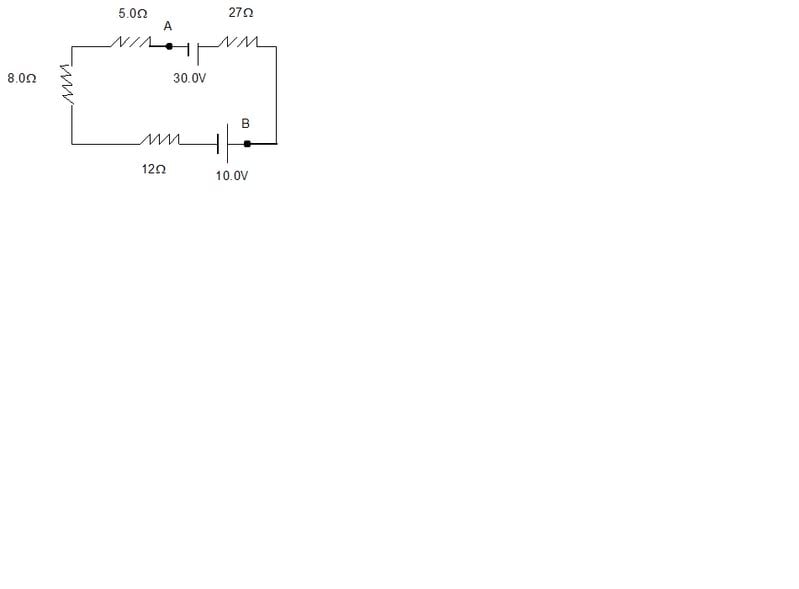# Determine the magnitude of the current in the circuit

This is a problem I had for homework. I want to make sure I did it correctly.

http://i90.photobucket.com/albums/k256/dedra73/circuit-2.jpg(a) Determine the magnitude of the current in the circuit and
I = (30-10)V / (27+5+8+12)Ω
=20V/52Ω
=.3846A

(b) The magnitude of the voltage between the points labeled A and B.
Vab = 30V - .3846A * 27Ω
= 30V- 10.3842V
= +19.6158V

(c) State which point, A or B, is at the higher potential.
Point B is 19.6158V higher than A

Last edited by a moderator: